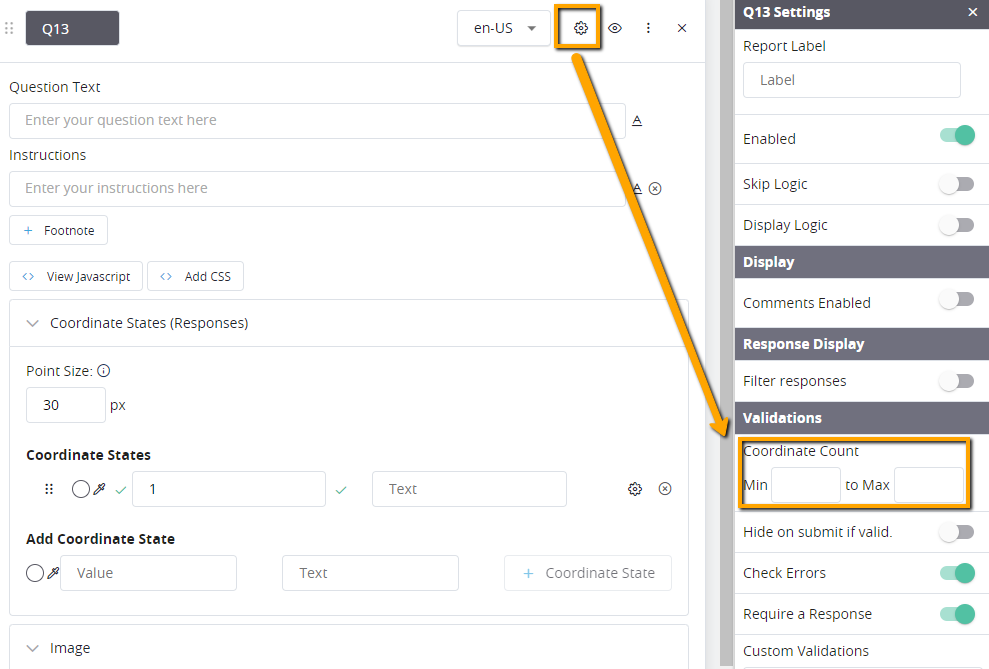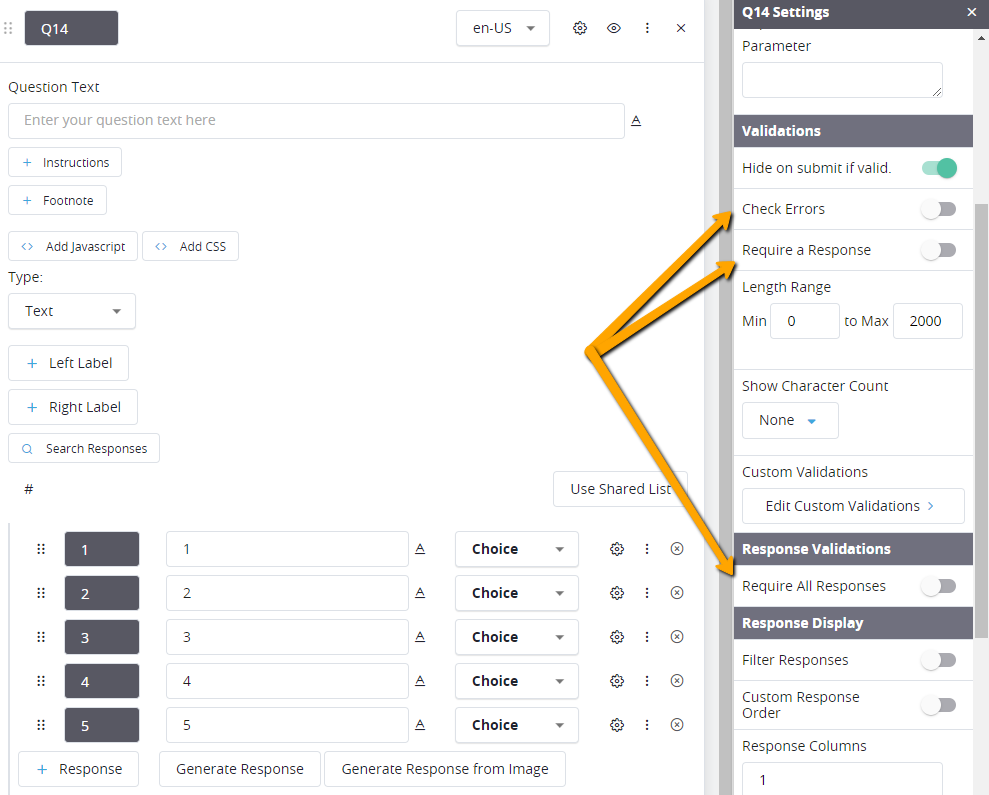# Coordinate Tracker: Re-display the Image with a Random Coordinate

This article will show you how to re-display a Coordinate Tracker with a random coordinate

### Background

You may want to ask a respondent to evaluate a 'Coordinate Tracker' exercise and then ask followup question tied to specific coordinates. This article will show you how to do so.

There are a few different parts to the process. Follow the steps below to accomplish this.

### Part 1 - Program the Coordinate Tracker

2. Add the following to the '+ Javascript' field
• See article: Adding JavaScript and/or CSS to a Question
• In 'Part 2' you will have to add a corresponding text question to hold the coordinates to be displayed. You will need to update the code below with that question number. It is towards the bottom of this code.
```var scaling;
var currentX, currentY;
setTimeout(function() {
scaling = parseFloat(jQuery(".cm-coordinate-tracker-image").css("width")) / parseFloat(jQuery(".cm-coordinate-tracker").attr("data-image-width"));
}, 500);

jQuery(".cm-coordinate-tracker-image-container img").click(function() {
setTimeout(function() {
populateCoordinates();
attachEvents();
}, 500)
if (jQuery(".cm-coordinate").length == 5 || jQuery("input.cm-exclusiveResponse").prop("checked")) {
attachEvents();
return false;
}
});

function populateCoordinates() {
jQuery(".cm-response-group input:text").val("");
jQuery.each(jQuery(".cm-coordinate"), function (index, element) {
currentX = parseFloat(jQuery(element).css("left")) + parseFloat(jQuery(element).find(".cm-coordinate-button").css("width")) / 2;
currentY = parseFloat(jQuery(element).css("top")) + parseFloat(jQuery(element).find(".cm-coordinate-button").css("height")) / 2;
jQuery(".cm-response-group input:text").eq(index).val(currentX/scaling + "|" + currentY/scaling);
});
}

function attachEvents() {
jQuery(".cm-coordinate-remove-button").click(function() {
setTimeout(function() {
populateCoordinates();
}, 500)
});
}

var qName = "Q2";  /* UPDATE Q2 TO THE TEXT QUESTION NUMBER */
jQuery("input.cm-exclusiveResponse").change(function () {
if (jQuery(this).prop("checked")) {
jQuery("div[data-qnum=" + qName + "] input:text").val("");
}
});
```
3. Access the 'Overall Question Settings'
4. Update the 'Coordinate Count' min and max fields to control how many possible coordinates can be added.### Part 2 - Save the 'Coordinates' to Re-display

1. On the same page as your 'Coordinate Tracker', program a 'Text Question'
2. Program as the same amount of responses as you have a max in Part 1 above. If your max is 5 then you will have 5 responses here. They can be blank responses.
3. Access the 'Overall Question Settings'
• See article: Overall Question Settings
• Add skip logic if TEST="N"
• Turn OFF the 'Check Errors', 'Require a Response' and the 'Require all Responses'### Part 3 - Randomly Pick a Coordinate to Re-display

1. Program a 'Concept' again with the max number of coordinates programmed in Part 1
• In the 'Count Range' update the number of coordinates you want to ask about going foward. So if you have up to 5 total in the 'Coordinate Tracker' question and you want ask follow ups for up to 2 of the total 5, you will make this 2.
• In the logic block, you will leave it as 'Always' but in the block itself you will add the following logic statement. Make sure to update it to be the same question number as the 'Text Fields Question' you programmed in Part 2 above.
• TRY ( "Q2_" . X ) != ""

### Part 4 - Evaluate the 'Concept'

1. Add a 'Logic Block Page' after the page with the 'Coordinate Tracker' and 'Text Fields Question'
• See article: Adding a Logic Block
• Here you will always 'Evaluate Concept' and select the 'Concept' you created in Part 3

### Part 5 - Re-display the 'Coordinate Tracker'

1. Program your followup question. If there are multiple, you will have to do the same thing for each one.
2. In the 'Source Code Editor' field of the area you want to re-display the image, add the following code:
• See article: Source Code Editor
• Update the image url to be the same one you used in the 'Coordinate Tracker' in Part 1 above
```<div id="imgContainer"><img class="fr-dib" src="https://YOUR_IMAGE_HERE.jpg">
<div id="coordContainer"> </div>
<br>
```
3. Add the following code to the '+ Javascript' field and update the code in line 1 to match your programming
1. Update the 'Q2' to match the question number you named your 'Text Fields' question from Part 2 above
2. Update the 'COORDINATES' to match the name of your 'Concept' from Part 3 above
3. *If you are going to show more than one, update the '1' to '2' for the second and '3' for the third and so on
```var pickedPoint = '[[Q2:(AT(COORDINATES,1))]]';
var coordinatesArray = pickedPoint.split("|");

var tempWidth = 0;
var naturalImage = new Image();
naturalImage.src = jQuery("#imgContainer img").attr("src");
tempWidth = this.width;
};

setTimeout(function(){
var scaling = tempWidth / parseFloat(jQuery("#imgContainer img").css("width"))
console.log(scaling);
jQuery("#coordContainer").append('<div class="cm-coordinateAdded" data-state="1" style="width: 30px; height: 30px; left: ' + ((coordinatesArray/scaling) - 15) + 'px; top: ' + ((coordinatesArray/scaling) - 15) + 'px;"></div>');
},200);
```
4. Add the following code to the '+CSS' field
1. Update the code below to match the coordinate color in the 'Coordinate Tracker' question
```#coordContainer {
/*	position: relative; */
top: 0;
left: 0;
}

#imgContainer {
position: relative;
}